## Sample Test Problems

1. A particle is confined to a box of lengthin one dimension. It is initially in the ground state. Suddenly, one wall of the box is moved outward making a new box of length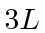. What is the probability that the particle is in the ground state of the new box? You may find it useful to know that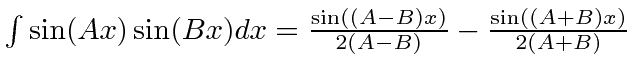2. A particle of massis in a 1 dimensional box of length L. The particle is in the ground state. The size of the box is suddenly (symmetrically) expanded to length 3L. Find the probability for the particle to be in the ground state of the new potential. (Your answer may include an integral which you need not evaluate.) Find the probability to be in the first excited state of the new potential.
3. Two degenerate eigenfunctions of the Hamiltonian are properly normalized and have the following properties.What are the properly normalized states that are eigenfunctions of H and P? What are their energies?
4. Find the first (lowest) three Energy eigenstates for a particle localized in a box such that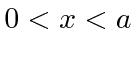. That is, the potential is zero inside the box and infinite outside. State the boundary conditions and show that your solutions satisfy them. Normalize the solutions to represent one particle in the box.
5. A particle is in the first excited state of a box of length. What is that state? Now one wall of the box is suddenly moved outward so that the new box has length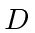. What is the probability for the particle to be in the ground state of the new box? What is the probability for the particle to be in the first excited state of the new box?
6. * Assume that. What is? What is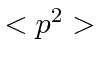? What is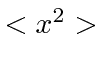?
7. For a free particle, the Hamiltonian operator H is given by. Find the functions,, which are eigenfunction of both the Hamiltonian and of. Write the eigenfunction that has energy eigenvalueand momentum eigenvalue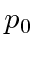. Now write the corresponding eigenfunctions in momentum space.
8. * A particle of massis in a 1 dimensional box of length L. The particle is in the ground state. A measurement is made of the particle's momentum. Find the probability that the value measured is betweenand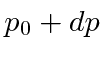.
9. A particle of massis in a constant potentialfor all x. What two operators commute with the Hamiltonian and can therefore be made constants of the motion? Since these two operators do not commute with each other, there must be two ways to write the energy eigenfunctions, one corresponding to each commuting operator. Write down these two forms of the eigenfunctions of the Hamiltonian that are also eigenfunctions of these two operators.
10. A particle is confined to a "box" in one dimension. That is the potential is zero forbetween 0 and, and the potential is infinite forless than zero orgreater than.
a)
Give an expression for the eigenfunctions of the Hamiltonian operator. These are the time independent solutions of this problem. (Hint: Real functions will be simplest to use here.)
b)
Assume that a particle is in the ground state of this box. Now one wall of the box is suddenly moved from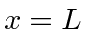to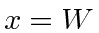where. What is the probability that the particle is found in the ground state of the new potential? (You may leave your answer in the form containing a clearly specified integral.)
11. A particle of massis in a 1 dimensional box of length. The particle is in the ground state. The size of the box is suddenly expanded to length. Find the probability for the particle to be in the ground state of the new potential. (Your answer may include an integral which you need not evaluate.) Find the probability to be in the first excited state of the new potential.
Jim Branson 2013-04-22